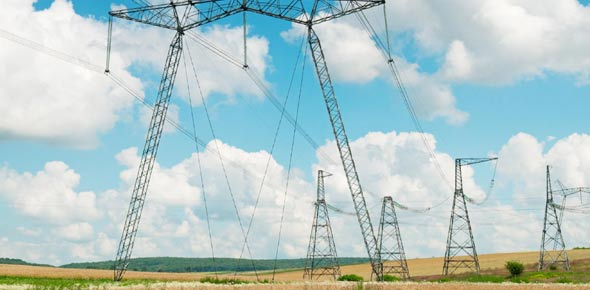# Chapter 11_basics Of Electricity

32 Questions | Total Attempts: 415SettingsElectricity as we have already covered in the previous classes flows easily in some materials as compared to others. What else do you remember from our basic introduction to electricity? Give the quiz below a try and highlight the questions that you fail to answer on your part. All the best!

• 1.
Electricity is descired as a form of
• A.

Energy

• B.

Movement

• C.

Negativity

• D.

Shortness

• 2.
The flow on electricity along a conductor is called a(n):
• A.

Electric charge

• B.

Electric current

• C.

Swirl

• D.

Spark

• 3.
Metals used in electric wiring and motors are materials that are considered good electricity
• A.

Current

• B.

Conductors

• C.

Wattage

• D.

Ohms

• 4.
Electric wires are usually covered with a rubber substance that is used as an insulator or:
• A.

Nonconductor

• B.

Mechanical

• C.

Current

• D.

Voltage

• 5.
The path of electricity from the generating source through conductors and back to the original source is called a (n):
• A.

Electric charge

• B.

Alternating charge

• C.

Insulator

• D.

Complete circuit

• 6.
Electric current that is a constant, even-flowing and travels in one direction is:
• A.

Direct current

• B.

Direct volt

• C.

Converter

• D.

Active current

• 7.
The device that changes direct current to alternating current is a(n):
• A.

Convex

• B.

Converter

• C.

Voltage

• D.

Ohm

• 8.
A rapid and interrupted current that flows in one direction then in the opposite direction is:
• A.

Rectifer current

• B.

Alternating current

• C.

Active current

• D.

Direct current

• 9.
The unit that measures the pressure or force that pushes the flow of electrons through a conductor is a (n)
• A.

Amp

• B.

Rectifer

• C.

Apparatus

• D.

Volt

• 10.
The unit that measures the amount of electric current is a
• A.

Battery

• B.

Volt

• C.

Ampere

• D.

Charger

• 11.
The current that is used for facial and scalp treatments is measured in
• A.

Millaperes

• B.

Direct current

• C.

Wattage

• D.

Voltage

• 12.
The unit that measures how much electric energy is being used in one second is a
• A.

Ohm

• B.

Watt

• C.

Amp

• D.

Volt

• 13.
The device that prevents the excessive current from passing through a circuit is
• A.

Fuse

• B.

Battery

• C.

Kilowatt

• D.

Ampere

• 14.
A switch that automatically unterrups or shuts off an electric current at the first indication of an overload is a
• A.

Voltage regulator

• B.

Ampere current

• C.

Circuit breaker

• D.

Battery charger

• 15.
An important way of promoting electrical safety is the principle of
• A.

Grounding

• B.

Connections

• C.

Low frequency

• D.

Visble light

• 16.
An applicator that is used for directing the electric current from the machine to the clientsskin in called the
• A.

Steamer

• B.

Anaphoresis

• C.

Polarity

• D.

Electode

• 17.
The postitive electode of an electrotherapy device is called
• A.

Frequency

• B.

Anode

• C.

Vaporizer

• D.

Accelerating

• 18.
The negative electrode of an electrotherapy device is called the
• A.

Cathode

• B.

Electron

• C.

Carbon

• D.

Therapeutic

• 19.
The most commonly used modality that is a constant and direct current is a
• A.

Indirect current

• B.

Active current

• C.

Galanvic current

• D.

Telsa current

• 20.
The process of introducing water soluble products into the skin with the use of electric current is called:
• A.

Chemical reaction

• B.

Inactive reaction

• C.

Iontophoesis

• D.

Electrodes

• 21.
A process that forces liquids into the tissues from the negative toward the positive pole is called
• A.

Anaphoresis

• B.

Deincrustation

• C.

Iontophoesis

• D.

Thereputic light

• 22.
A thermal or heat-producing current with a high rate of oscillation that is used for scalp and facial treatments is:
• A.

Telsa current

• B.

Direct current

• C.

Alternating current

• D.

Infrared current

• 23.
Visable light, the part of the electromagnetic radiation spectrum that we can see, makes up what percent of natural sunlight
• A.

60%

• B.

25%

• C.

35%

• D.

50%

• 24.
The longwavelengths that penetrate the deepest and produce the most heat are
• A.

Blue rays

• B.

Utlraviolet rays

• C.

Natural rays

• D.

Infrared rays

• 25.
The term used for measuring electrical current
• A.

Watt

• B.

Amp

• C.

Ohm

• D.

Volt

Related TopicsBack to top